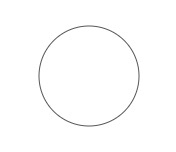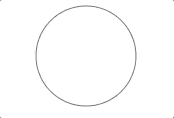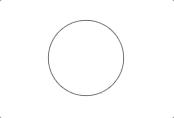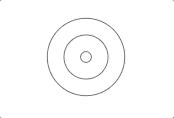有个项目需要在某个坐标显示一个声波扩散(不知道这个表达对不对)的动画。这种需求一般做法有几种，一种做成gif图片，然后贴上去，一种是用html+css3完成，要么就是画上去，这画又分两种，一种是Canvas画布来画，另一种用svg来画。

制作成gif图片往上贴，如果有ui负责做图，这对于前端来说是最省事的方法，只要贴上去就完了，不过这种方法有一个缺点，你能控制的有限，如果要用另一种颜色，或者改变圈数，只能重新制作一张图片，重复替换，而且动画的重复次数也不好控制。pass

html+css3完成，这个简单，不过效果有限，看这里。pass

画上去分两种，一种canvas画布，一种svg画布，关于两者的优劣比较不是这里的主体，在这里不讨论。而且对于小需求来说，两者的资源消耗差异其实也可以忽略不计。关于canvas的声波扩散实现，看这里

好吧，言归正传，既然选择了方向，那便开始。

首先，写一个svg画布，宽高200px

<svg id="svgView" width="200" height="200"></svg>

然后在里面画一个圆。

<svg id="svgView" width="200" height="200">
<circle cx="80" cy="80" r="50" stroke="#555" fill-opacity="0"></circle>
</svg>

这里circle表示一个圆，圆心坐标以svgView画布左上角为原点，向右偏移cx个单位，向下偏移cy个单位，r为圆半径，stroke表示圆边框填充颜色，fill表示圆内填充颜色，fill-opacity表示圆内填充透明度。完整API

效果：接下来添加动画效果。这里用到了svg的SMIL animation

<svg id="svgDom" width="200" height="200">
<circle cx="80" cy="80" r="50" stroke="#555" fill-opacity="0">
<animate attributeName="r" begin="0" from="0" to="50" dur="2s"></animate>
</circle>
</svg>

attributeName表示动画进行改变的是图像的什么属性；begin为开始时间，单位为秒；from为开始值，to为目标值，其中from可选，dur表示动画持续时间。如果不添加repeatCount属性，表示动画执行一次便结束。

效果：我们的图片是需要不停的扩散的，于是添加repeatCount属性，让其无限循环。

<svg id="svgDom" width="200" height="200">
<circle cx="80" cy="80" r="50" stroke="#555" fill-opacity="0">
<animate attributeName="r" begin="0" from="0" to="50" dur="2s" repeatCount="indefinite"></animate>
</circle>
</svg>

indefiniter表示无限循环至电脑死机。

效果：设计里，我们的扩散环有三环，然后每环延迟0.5s释放，当释放至最大消失，由此循环。

<svg id="svgDom" width="200" height="200">
<circle cx="80" cy="80" r="50" stroke="#555" fill-opacity="0">
<animate attributeName="r" id="ani1" begin="0" from="0" to="50" dur="1.5s" repeatCount="indefinite"></animate>
</circle>
<circle cx="80" cy="80" r="50" stroke="#555" fill-opacity="0">
<animate attributeName="r" begin="ani1.begin + 0.5s" from="0" to="50" dur="1.5s" repeatCount="indefinite"></animate>
</circle>
<circle cx="80" cy="80" r="50" stroke="#555" fill-opacity="0">
<animate attributeName="r" begin="ani1.begin + 1s" from="0" to="50" dur="1.5s" repeatCount="indefinite"></animate>
</circle>
</svg>

其中，begin为动画开始时间，可以设置具体的时间秒数，也可以根据animate 的id值，来获取该id的animate动画开始结束时间，然后添加偏移数据，来控制多个动画的依序进行。

效果：到这里，其实已经达到效果了，不过我们可以进一步添加一个效果，让圆环在扩散过程中慢慢消失。并且将圆环宽度设置为5px。

<svg id="svgDom" width="200" height="200">
<circle cx="80" cy="80" r="0" stroke="#555" stroke-width="5" fill-opacity="0">
<animate attributeName="r" id="ani1" begin="0" from="0" to="50" dur="1.5s" repeatCount="indefinite"></animate>
<animate attributeName="opacity" begin="0" from="1" to="0" dur="1.5s" repeatCount="indefinite"></animate>
</circle>
<circle cx="80" cy="80" r="0" stroke="#555" stroke-width="5" fill-opacity="0">
<animate attributeName="r" begin="ani1.begin + 0.5s" from="0" to="50" dur="1.5s" repeatCount="indefinite"></animate>
<animate attributeName="opacity" begin="ani1.begin + 0.5s" from="1" to="0" dur="1.5s" repeatCount="indefinite"></animate>
</circle>
<circle cx="80" cy="80" r="0" stroke="#555" stroke-width="5" fill-opacity="0">
<animate attributeName="r" begin="ani1.begin + 1s" from="0" to="50" dur="1.5s" repeatCount="indefinite"></animate>
<animate attributeName="opacity" begin="ani1.begin + 1s" from="1" to="0" dur="1.5s" repeatCount="indefinite"></animate>
</circle>
</svg>

最终效果：到这里，这个svg版声纹扩散动画已经做完了。

posted on 2018-04-03 17:29  linxiong  阅读(1284)  评论(0编辑  收藏